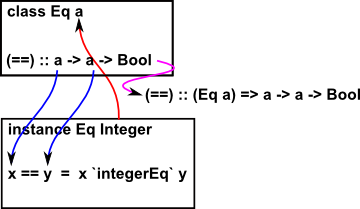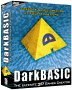### Values and Types

A value may be represented with its type signature, by using the :: symbol, or its type may be automatically inferred.

 myValue :: MyType ^ values are: first class first letter not capitalised ^ has type ^ values are: not first class first letter capitalised

### Defining Functions

A function, in this case 'inc' may have a type signature and a definition:

In file (in module) In console
inc :: Integer -> Integer   type signature of function
inc n = n + 1 let inc n = n + 1 declaration of function

Functions can only be defined in a limited way from console, using 'let' keyword. For more complicated fuctions it is necessay to put in a file.

 inc :: Integer -> Integer type signature of function inc n = n + 1 declaration of function

#### Anonymous Functions

 example: lambda function for 'square' (\x -> x*x) ```Prelude> (\x -> x*x) 3 9 Prelude> ```

The \ character is intended to represent the λ symbol.

### Defining Data

Types defined in prelude (effectivly built in types):

Type Example
Numeric types (see table below) 3
Boolean True
Char 'c'
String (same as [Char]) "ex"

Numeric Classes
Num Real Integral Fractional Floating RealFrac RealFloat
Int
Integer 3
Float exp(6.8)
Double
Rational 6/7

#### User Types

The 'data' keyword allows us to define a type constructor with its corresponding data constructors.

An algebraic user type uses a '|' symbol to provide alternative value constructors. This can be read as 'or'. It provides an equivalent of enumeration in other languages.

data Type Constructor = Data Constuctor
algebraic user type:
data
Bool
=
False | True

^
nullary type constructor

^
nullary data constructors
(enumerated types
disjoint union of sum types)

polymorphic user type:
data
Point a
=
Pt a a

^
type constructor

^
value data constructor
(tuple otherwise known as
polymorphic type - 'a' can represent any type)

list: (how it would be defined if not built in)
data
[a]
=
[] | a : [a] deriving (Eq, Ord)

^
List of type a (also written [] a)
.

where:
a : [a] means first:[remainder]
so : is defined by:
(:) :: a -> [a] -> [a]

tree:
data
Tree a
=
Leaf a | Branch (Tree a) (Tree a)
which implies the following types:

Branch
::
Tree a -> Tree a -> Tree a

Leaf
::
a -> Tree

#### type synonyms

We can use an alternative name for a type by using the 'type' keyword. This is purely to make the code more readable.

type myID = Int
type String = [char]

#### newtype

This creates a new type from an existing type, it is like renaming an existing type, it hides this derivation and gives the type a new identity.

#### Variables

Variables in Haskell, and other pure functional languages, work differently than variables in imperative languages. A pure functional language can't have a state so a variable cant actually vary, we can assign, say x, a value:

In file (in module) In console
x = 1 let x = 1

but if we then try to give it another value:

x = 2

this will assign another variable 'x' which may, or may not, generate an error.

Also this does not work:

x = x + 1

because its treated as being recursive.

 ```Prelude> let x = 1 Prelude> x 1 Prelude> let x = 2 Prelude> x 2 Prelude> let x = x + 1 Prelude> x ^CInterrupted. Prelude> ```

### Typeclasses

Haskell classes support polymorphism, overloading and inheritance, a class defines which operations can work on which inputs.Haskell classes are not objects, in that they do not have data, as that would be state information which is not allowed in a pure functional program.

 ```class MyCompare a where myEq,myNotEq :: a -> a -> Bool myEq x y = not (myNotEq x y)``` <-- define a class MyCompare <-- function signatures <-- boilerplate implementations

defining an instance of a class:

```instance MyCompare Int where
myEq x y = x==y```

In order to test this out I have put the following into a file:

 ```class MyCompare a where myEq,myNotEq :: a -> a -> Bool myEq x y = not (myNotEq x y) instance MyCompare Int where myEq x y = x==y myNotEq x y = x/=y```

 ```*Main> :load mycompare.hs [1 of 1] Compiling Main ( mycompare.hs, interpreted ) Ok, modules loaded: Main. *Main> :info myEq class MyCompare a where myEq :: a -> a -> Bool ... -- Defined at mycompare.hs:2:4-7 *Main> :type myEq myEq :: (MyCompare a) => a -> a -> Bool *Main>```

note that (MyCompare a) => means: for all types 'a' provided that 'a' is an instance of MyCompare.

### Lists & Tuples

type fixed length fixed
List yes no
Tuple no yes

### Lists

List values are represented by enclosing the values in square brackets seperated by commas:

['a','b','c']

List type also represented by putting in square brackets:

[Char]

In the following constructs the element 'a' is of polymorphic type. That is, lists can be defined of any other types.

This construct ':' appends to a list.

 a : [] ^ first element ^ append ^ remaining elements in this case empty list

List Operations and Functions
(++) :: [a] -> [a] -> [a] -- Defined in GHC.Base concatinating lists: a ++ b
head :: [a] -> a -- Defined in GHC.List

tail :: [a] -> [a] -- Defined in GHC.List
take :: Int -> [a] -> [a] -- Defined in GHC.List
drop :: Int -> [a] -> [a] -- Defined in GHC.List

last :: [a] -> a -- Defined in GHC.List
map :: (a -> b) -> [a] -> [b] -- Defined in GHC.Base

filter :: (a -> Bool) -> [a] -> [a] -- Defined in GHC.List
foldl :: (a -> b -> a) -> a -> [b] -> a -- Defined in GHC.List
foldr :: (a -> b -> b) -> b -> [a] -> b -- Defined in GHC.Base

List Comprehensions

 term Generators Guard [ f x x <- xs ] [(x,y) | x <- xs, y<- ys] [ y y | y <- xs , y

Arithmetic Sequence

 [1..10] [1,2,3,4,5,6,7,8,9,10] [1,3 .. 10] continue step size between first two elements [1,3,5,7,9] [1..3..] infinite sequence

### Tuples

Tuple Operations and Functions
fst
snd

## Pattern Matching

Pattern matching when calling functions:

'_' can be used as a wild card.

We can also pattern match on a algebraic value constructor.

• wiki
• Advanced Topics in Programming Languages Series: Parametric Polymorphism and the Girard-Reynolds Isomorphism. This talk is based on a series of papers by Philip Wadler, a principal designer of the Haskell programming language. Featured are a number of double-barreled names in computer science:

• Hindley-Milner (Strong typing without having to type the types)
• Curry-Howard (Isomorphism between types and theorems, terms and proofs)
• Girard-Reynolds (Isomorphism between types and terms in the presence of parametricity)
• For Djinn code see here.
• Parametricity

Where I can, I have put links to Amazon for books that are relevant to the subject, click on the appropriate country flag to get more details of the book or to buy it from them.Visual Basic .NET for Microsoft Platforms only.

Commercial Software Shop

Where I can, I have put links to Amazon for commercial software, not directly related to this site, but related to the subject being discussed, click on the appropriate country flag to get more details of the software or to buy it from them.Dark Basic Professional Edition - It is better to get this professional editionThis is a version of basic designed for building games, for example to rotate a cube you might do the following: make object cube 1,100 for x=1 to 360 rotate object 1,x,x,0 next x

This site may have errors. Don't use for critical systems.